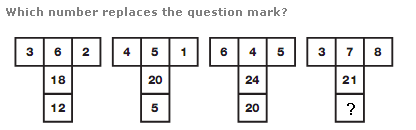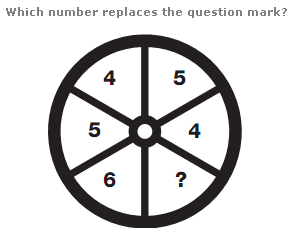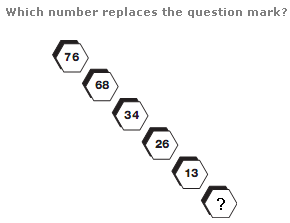# Puzzles - Number puzzles

### Exercise :: Number puzzlesAnswer : 56 Explanation : In each diagram, multiply the left hand number by the upper central number to give the central number one line down, and multiply the right hand number by the upper central number to give the lower central number.Answer : 3 Explanation : Split the circle in half vertically, and take pairs of digits from corresponding segments of the left and right hand halves of the circle. Reading these pairs of digits as 2 digit numbers, these numbers follow the sequence of multiples of 9, from 45 to 63.Answer : 5 Explanation : As you go down, alternately subtract 8 to give the next number, then divide by 2. Repeat this pattern.